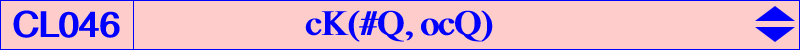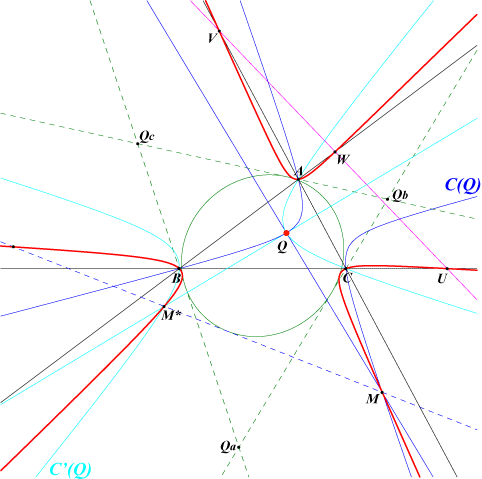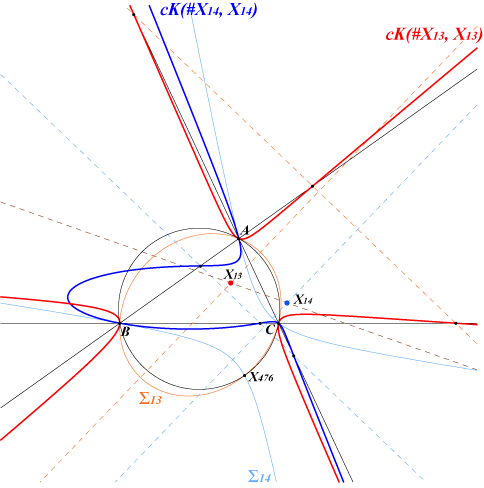Let Q be a fixed finite point not lying on the sidelines of ABC and C(Q) a circum-conic passing through Q. The normal at Q to C(Q) meets C(Q) again at M. The locus of M is the cubic K = cK(#Q, ocQ) = nK(Q^2, ocQ, Q), where ocQ is the orthocorrespondent of Q. Hence, the cubic K is a nodal non-pivotal isocubic with node Q which is always an isolated point on the curve. K meets the sidelines of ABC at U, V, W lying on the orthotransversal of ocQ (U is the intersection of BC and the perpendicular at Q to QA). Let C'(Q) be the circum-conic passing through Q and orthogonal at Q to C(Q). The normal at Q to C'(Q) (i.e. the tangent at Q to C(Q)) meets C'(Q) at M*, the Q^2-isoconjugate of M, which obviously lies on K. Moreover, the line MM* envelopes the pivotal conic of K. This is the conic inscribed in the anticevian triangle QaQbQc of Q which is tangent to the lines AU, BV, CW. Amazingly, Q is one focus of this pivotal conic and the corresponding directrix is the line containing the three real inflexion points of the cubic. The trilinear polars of Q and ocQ meet at a point on this directrix. These three lines are parallel when Q lies on Q003, the Euler-Morley quintic. Note that if U1 = BV /\ CW, V1 = CW /\ AU, W1 = AU /\ BV then the points Qa, Qb, Qc, U1, B1, C1, Q, ocQ lie on a same conic.Special cases 1. The line UVW is the line at infinity if and only if ocQ is the centroid G i.e. if and only if Q is the orthocenter H. This gives the cubic cK(#X4, X2) = K406. The pivotal conic is the MacBeath conic of the antimedial triangle. Its center is O. 2. When Q is a Fermat point X(13) or X(14), ocQ = Q hence the corresponding cubics are cK(#X13, X13) and cK(#X14, X14). In both cases, the pivotal conic and the contact conic coincide. These conics are the Simmons circum-conics ∑13 and ∑14.3. When Q is an in/excenter, the cubic is isogonal. For example, with Q = X(1), we obtain K407 = cK(#X1, X57) = nK(X6, X57, X1). The pivotal conic is the MacBeath conic of the excentral triangle. 4. When Q is the centroid G (or a vertex of the antimedial triangle), the cubic is isotomic. For example, with Q = X(2), we obtain K408 = cK(#X2, X1992) = nK(X2, X1992, X2). The pivotal conic is the Lemoine ellipse of the antimedial triangle. 5. When Q is the circumcenter O, we obtain K658 = cK(#X3, X1993).